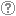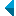# Iskanje po repozitorijuIskalni niz: išči po NaslovAvtorOpisKljučne besedePolno besediloLeto izida INALIIN NE išči po NaslovAvtorOpisKljučne besedePolno besediloLeto izida INALIIN NE išči po NaslovAvtorOpisKljučne besedePolno besediloLeto izida INALIIN NE išči po NaslovAvtorOpisKljučne besedePolno besediloLeto izida Vrsta gradiva: Vse vrste gradiv Habilitacijsko delo (m4) Višješolska diplomska naloga (m6) Specialistično delo (m3) Diplomsko delo * (dip) Magistrsko delo * (mag) Doktorska disertacija * (dok) Raziskovalni podatki ali korpus (data) * po starem in bolonjskem študiju Jezik: Vsi jezikiSlovenski jezikAngleški jezikNemški jezikHrvaški jezikSrbski jezikBolgarski jezikBosanski jezikČeški jezikFinski jezikFrancoski jezikItalijanski jezikJaponski jezikLitvanski jezikMadžarski jezikNemški jezik (Avstrija)Norveški jezikPoljski jezikRuski jezikSlovaški jezikSrbski jezik (cirilica)Španski jezikŠvedski jezikTurški jezikNeznan jezik Išči po: RUP    FAMNIT - Fakulteta za matematiko, naravoslovje in informacijske tehnologije    FHŠ - Fakulteta za humanistične študije    FM - Fakulteta za management    FTŠ Turistica - Fakulteta za turistične študije - Turistica    FVZ - Fakulteta za vede o zdravju    IAM - Inštitut Andrej Marušič    PEF - Pedagoška fakulteta    UPR - Univerza na Primorskem    ZUP - Založba Univerze na PrimorskemCOBISS    Fakulteta za humanistične študije, Koper    Fakulteta za management Koper in Pedagoška fakulteta Koper    Fakulteta za vede o zdravju, Izola    Knjižnica za tehniko, medicino in naravoslovje, Koper    Turistica, Portorož    Znanstveno-raziskovalno središče Koper Opcije: Prikaži samo zadetke s polnim besedilom Ponastavi

 1 - 10 / 19121.Rank-permutable additive mappingsAleksandr Èmilevič Guterman, Anna A. Alieva, Bojan Kuzma, 2006, izvirni znanstveni članekOpis: Let ▫$\sigma$▫ be a fixed non-identical permutation on ▫$k$▫ elements. Additive bijections ▫$T$▫ on the matrix algebra ▫$M_n(\mathbb{F})$▫ over a field ▫$\mathbb{F}$▫ of characteristic zero, with the property that ▫$\rm{rk} (A_1...A_k) = \rm{rk} (A_{\sigma(1)}...A_{\sigma(k)})$▫ implies the same condition on the ▫$T$▫ images, are characterized. It is also shown that the surjectivity assumption can be relaxed, if this property is preserved in both directions.Najdeno v: ključnih besedahPovzetek najdenega: ...Let ▫$\sigma$▫ be a fixed non-identical permutation on ▫$k$▫ elements. Additive bijections ▫$T$▫ on... Ključne besede: mathematics, linearna algebra, matrix algebra, rank, permutation, additive preserversObjavljeno: 15.10.2013; Ogledov: 2632; Prenosov: 85Polno besedilo (0,00 KB) 2.Classification of 2-arc-transitive dihedrantsAleksander Malnič, Shao Fei Du, Dragan Marušič, 2008, izvirni znanstveni članekOpis: A complete classification of 2-arc-transitive dihedrants, that is, Cayley graphs of dihedral groups is given, thus completing the study of these graphs initiated by the third author in [D. Marušič, On 2-arc-transitivity of Cayley graphs, J. Combin. Theory Ser. B 87 (2003) 162-196]. The list consists of the following graphs: (i) cycles ▫$C_{2n},\; n \ge 3$▫; (ii) complete graphs ▫$K_{2n}, \; n \ge 3$▫; (iii) complete bipartite graphs ▫$K_{n,n}, \; n \ge 3$▫; (iv) complete bipartite graphs minus a matching ▫$K_{n,n} - nK_2, \; n \ge 3$▫; (v) incidence and nonincidence graphs ▫$B(H_{11})$▫ and ▫$B'(H_{11})$▫ of the Hadamard design on 11 points; (vi) incidence and nonincidence graphs ▫$B(PG(d,q))$▫ and ▫$B'(PG(d,q))$▫, with ▫$d \ge 2$▫ and ▫$q$▫ a prime power, of projective spaces; (vii) and an infinite family of regular ▫${\mathbb{Z}}_d$▫-covers ▫$K_{q+1}^{2d}$▫ of ▫$K_{q+1, q+1} - (q+1)K_2$▫, where ▫$q \ge 3$▫ is an odd prime power and ▫$d$▫ is a divisor of ▫$\frac{q-1}{2}$▫ and ▫$q-1$▫, respectively, depending on whether ▫$q \equiv 1 \pmod{4}$▫ or ▫$q \equiv 3 \pmod{4}$▫ obtained by identifying the vertex set of the base graph with two copies of the projective line ▫$PG(1,q)$▫, where the missing matching consists of all pairs of the form ▫$[i,i']$▫, ▫$i \in PG(1,q)$▫, and the edge ▫$[i,j']$▫ carries trivial voltage if ▫$i=\infty$▫ or ▫$j=\infty$▫, and carries voltage ▫$\bar{h} \in {\mathbb{Z}}_d$▫, the residue class of ▫$h \in {\mathbb{Z}}_d$▫, if and only if ▫$i-j = \theta^h$▫, where ▫$\theta$▫ generates the multiplicative group ▫${\mathbb{F}}_q^\ast$▫ of the Galois field ▫${\mathbb{F}}_q$▫.Najdeno v: ključnih besedahPovzetek najdenega: ...permutation group, imprimitive group, dihedral group, Cayley graph,... Ključne besede: permutation group, imprimitive group, dihedral group, Cayley graph, dihedrant, 2-Arc-transitive graphObjavljeno: 15.10.2013; Ogledov: 2773; Prenosov: 85Polno besedilo (0,00 KB) 3.Primitive bicirculant association schemes and a generalization of Wielandt's theoremDragan Marušič, István Kovács, Mikhail Muzychuk, 2010, izvirni znanstveni članekNajdeno v: ključnih besedahPovzetek najdenega: ...permutation group, association scheme, primitive association scheme, ... Ključne besede: permutation group, association scheme, primitive association schemeObjavljeno: 15.10.2013; Ogledov: 2612; Prenosov: 123Polno besedilo (0,00 KB) 4.Decomposition of skew-morphisms of cyclic groupsIstván Kovács, Roman Nedela, 2011, izvirni znanstveni članekOpis: A skew-morphism of a group ▫$H$▫ is a permutation ▫$\sigma$▫ of its elements fixing the identity such that for every ▫$x, y \in H$▫ there exists an integer ▫$k$▫ such that ▫$\sigma (xy) = \sigma (x)\sigma k(y)$▫. It follows that group automorphisms are particular skew-morphisms. Skew-morphisms appear naturally in investigations of maps on surfaces with high degree of symmetry, namely, they are closely related to regular Cayley maps and to regular embeddings of the complete bipartite graphs. The aim of this paper is to investigate skew-morphisms of cyclic groups in the context of the associated Schur rings. We prove the following decomposition theorem about skew-morphisms of cyclic groups ▫$\mathbb Z_n$▫: if ▫$n = n_{1}n_{2}$▫ such that ▫$(n_{1}n_{2}) = 1$▫, and ▫$(n_{1}, \varphi (n_{2})) = (\varphi (n_{1}), n_{2}) = 1$▫ (▫$\varphi$▫ denotes Euler's function) then all skew-morphisms ▫$\sigma$▫ of ▫$\mathbb Z_n$▫ are obtained as ▫$\sigma = \sigma_1 \times \sigma_2$▫, where ▫$\sigma_i$▫ are skew-morphisms of ▫$\mathbb Z_{n_i}, \; i = 1, 2$▫. As a consequence we obtain the following result: All skew-morphisms of ▫$\mathbb Z_n$▫ are automorphisms of ▫$\mathbb Z_n$▫ if and only if ▫$n = 4$▫ or ▫$(n, \varphi(n)) = 1$▫.Najdeno v: ključnih besedahPovzetek najdenega: ...skew-morphism of a group ▫$H$▫ is a permutation ▫$\sigma$▫ of its elements fixing the identity... Ključne besede: cyclic group, permutation group, skew-morphism, Schur ringObjavljeno: 15.10.2013; Ogledov: 3100; Prenosov: 104Polno besedilo (0,00 KB) 5.On cryptographically significant mappings over GF(2 [sup] n)Enes Pašalić, 2008, objavljeni znanstveni prispevek na konferenciOpis: In this paper we investigate the algebraic properties of important cryptographic primitives called substitution boxes (S-boxes). An S-box is a mapping that takes ▫$n$▫ binary inputs whose image is a binary ▫$m$▫-tuple; therefore it is represented as ▫$F:\text{GF}(2)^n \rightarrow \text{GF}(2)^m$▫. One of the most important cryptographic applications is the case ▫$n = m$▫, thus the S-box may be viewed as a function over ▫$\text{GF}(2^n)$▫. We show that certain classes of functions over ▫$\text{GF}(2^n)$▫ do not possess a cryptographic property known as APN (AlmostPerfect Nonlinear) permutations. On the other hand, when ▫$n$▫ is odd, an infinite class of APN permutations may be derived in a recursive manner, that is starting with a specific APN permutation on ▫$\text{GF}(2^k), k$▫ odd, APN permutations are derived over ▫$\text{GF}(2^{k+2i})$▫ for any ▫$i \geq 1$▫. Some theoretical results related to permutation polynomials and algebraic properties of the functions in the ring ▫$\text{GF}(q)[x,y]$▫ are also presented. For sparse polynomials over the field ▫$\text{GF}(2^n)$▫, an efficient algorithm for finding low degree I/O equations is proposed.Najdeno v: ključnih besedahPovzetek najdenega: ...cryptographic property known as APN (AlmostPerfect Nonlinear) permutations. On the other hand, when ▫$n$▫ is... Ključne besede: cryptoanalysis, cryptography, permutation polynomials, power mappings, APN functions, S-box, CCZ-equivalence, algebraic propertiesObjavljeno: 15.10.2013; Ogledov: 2485; Prenosov: 68Polno besedilo (0,00 KB) 6.Distance-transitive graphs admit semiregular automorphismsKlavdija Kutnar, Primož Šparl, 2010, izvirni znanstveni članekOpis: A distance-transitive graph is a graph in which for every two ordered pairs ofvertices ▫$(u,v)$▫ and ▫$(u',v')$▫ such that the distance between ▫$u$▫ and ▫$v$▫ is equal to the distance between ▫$u'$▫ and ▫$v'$▫ there exists an automorphism of the graph mapping ▫$u$▫ to ▫$u'$▫ and ▫$v$▫ to ▫$v'$▫. A semiregular element of a permutation group is anon-identity element having all cycles of equal length in its cycle decomposition. It is shown that every distance-transitive graph admits a semiregular automorphism.Najdeno v: ključnih besedahPovzetek najdenega: ...to ▫$v'$▫. A semiregular element of a permutation group is anon-identity element having all cycles... Ključne besede: distance-transitive graph, vertex-transitive graph, semiregular automorphism, permutation groupObjavljeno: 15.10.2013; Ogledov: 2716; Prenosov: 93Polno besedilo (0,00 KB) 7.Cubic symmetric graphs via odd automorphisms, 60th Birthday Lecture Series, Department of Mathematics, University of Auckland, New Zealand, 10 September 2015Klavdija Kutnar, 2015, predavanje na tuji univerziNajdeno v: ključnih besedahPovzetek najdenega: ...cubic graph, symmetric, automorphism, odd permutation, ... Ključne besede: cubic graph, symmetric, automorphism, odd permutationObjavljeno: 15.10.2015; Ogledov: 1944; Prenosov: 20Polno besedilo (0,00 KB) 8.Minimal normal subgroups of transitive permutation groups of square-free degreeAleksander Malnič, Edward Dobson, Dragan Marušič, Lewis A. Nowitz, 2007, izvirni znanstveni članekOpis: It is shown that a minimal normal subgroup of a transitive permutation group of square-free degree in its induced action is simple and quasiprimitive, with three exceptions related to ▫$A_5$▫, ▫$A_7$▫, and PSL(2,29). Moreover, it is shown that a minimal normal subgroup of a 2-closed permutation group of square-free degree in its induced action is simple. As an almost immediate consequence, it follows that a 2-closed transitive permutation group of square-free degree contains a semiregular element of prime order, thus giving a partial affirmative answer to the conjecture that all 2-closed transitive permutation groups contain such an element (see [D. Marušic, On vertex symmetric digraphs,Discrete Math. 36 (1981) 69-81; P.J. Cameron (Ed.), Problems from the fifteenth British combinatorial conference, Discrete Math. 167/168 (1997) 605-615]).Najdeno v: ključnih besedahPovzetek najdenega: Zadetek v naslovuKljučne besede: mathematics, graph theory, transitive permutation group, 2-closed group, square-free degree, semiregular automorphism, vertex-transitive graphObjavljeno: 03.04.2017; Ogledov: 1827; Prenosov: 85Polno besedilo (0,00 KB) 9.Semiregular automorphisms of vertex-transitive graphs of certain valenciesAleksander Malnič, Edward Dobson, Dragan Marušič, Lewis A. Nowitz, 2007, izvirni znanstveni članekOpis: It is shown that a vertex-transitive graph of valency ▫$p+1$▫, ▫$p$▫ a prime, admitting a transitive action of a ▫$\{2,p\}$▫-group, has a non-identity semiregular automorphism. As a consequence, it is proved that a quartic vertex-transitive graph has a non-identity semiregular automorphism, thus giving a partial affirmative answer to the conjecture that all vertex-transitive graphs have such an automorphism and, more generally, that all 2-closed transitive permutation groups contain such an element (see [D. Marušic, On vertex symmetric digraphs, Discrete Math. 36 (1981) 69-81; P.J. Cameron (Ed.), Problems from the Fifteenth British Combinatorial Conference, Discrete Math. 167/168 (1997) 605-615]).Najdeno v: ključnih besedahPovzetek najdenega: ...and, more generally, that all 2-closed transitive permutation groups contain such an element (see [D.... Ključne besede: mathematics, graph theory, transitive permutation group, 2-closed group, semiregular automorphism, vertex-transitive graphObjavljeno: 03.04.2017; Ogledov: 1884; Prenosov: 78Polno besedilo (0,00 KB) 10.Monomial isomorphisms of cyclic codesEdward Dobson, 2015, izvirni znanstveni članekNajdeno v: ključnih besedahPovzetek najdenega: ...Cyclic code, Monomial, Permutation, Isomorphism, Equivalent, ... Ključne besede: Cyclic code, Monomial, Permutation, Isomorphism, EquivalentObjavljeno: 03.04.2017; Ogledov: 1899; Prenosov: 129Polno besedilo (0,00 KB)
Iskanje izvedeno v 0 sek.
Na vrh